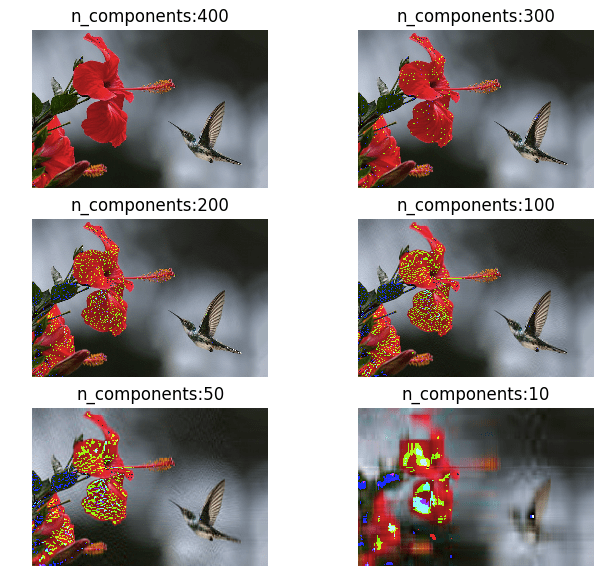# Mathematics## Converting Color Images to Grayscale using numpy and some Mathematics

An extremely magnified image at the end is just blocks of colors called pixels, where each pixel is formed by the combination of Red, Blue and Green, our primary colors. RGB color space or RGB color system, constructs all the colors from the combination of the intensities of Red, Green and Blue colors. The red, green and …## Mathematics of Principal component analysis

Principal component analysis is a method used to reduce the number of dimensions in a dataset without losing much information. It’s used in many fields such as face recognition and image compression, and is a common technique for finding patterns in data and also in the visualization of higher dimensional data. PCA is all about …

## Maths behind Polynomial regression

Polynomial regression is a process of finding a polynomial function that takes the form f( x ) = c0 + c1 x + c2 x2 ⋯ cn xn where n is the degree of the polynomial and c is a set of coefficients. Through polynomial regression we try to find an nth degree polynomial function which is the closest approximation of our data points. Below is a sample random dataset which has been regressed …

## Evaluating the fitness of a modal with a cost function

Previously we derived a simple linear regression modal for our Pizza price dataset. We built a modal that predicted a price of \$13.68 for a 12 inch pizza. When the same modal is used to predict the price of an 8 inch pizza, we get \$9.78 which is around \$0.78 more than the known price of \$9. …

## Math behind Linear Regression with Python code

Simple linear regression is a statistical method you can use to study relationships between two continuous (quantitative) variables: independent variable (x) – also referred to as predictor or explanatory variable dependant variable (y) – also referred to as response or outcome The goal of any regression model is to predict the value of y (dependant variable) based on the …

## Possibly the easiest explanation of differentiation and Integration in Calculus

I have read a lot of books on Calculus but “Calculus Made Easy” by Silvanus P. Thompson stands out as possibly the easiest book to read on this topic. It totally lives up to its name. The first chapter opens up with an impressive introduction on the difference between dx & ∫.  d which merely means …

## Understanding Binomial Distribution using Python

Binomial distribution is used to understand the probability of a particular outcome in repeated independent trials. The probability of a trial is either success or failure. The trials are independent as the outcome or the previous trial had no effect on the next trial, as happens in tossing of coins. If we flip a coin, it would either …

## Poisson distribution with Python

A Poisson distribution is the probability distribution of independent occurrences in an interval. Poisson distribution is used for count-based distributions where these events happen with a known average rate and independently of the time since the last event. For example, If the average number of cars that cross a particular street in a day is 25, …

## Graph Theory Basics – Part 1

A,B,C,D and E are vertices AB, BC, CD etc are edges the whole diagram is called a graph. The degree of a vertex is the number of edges with that vertex as an end-point; B is degree 2, written as deg(B) Graphs with no loops or multiple edges, such as the graph in 1, are called …

## Markow chain explained in simple words

Markow chain is a probabilistic process used to predict the next step based on the probabilities of the existing related states. Its called a chain because the probability of the next step is dependant on the other steps in the group. For example, if the weather is cloudy then its highly likely that it might rain (The next …